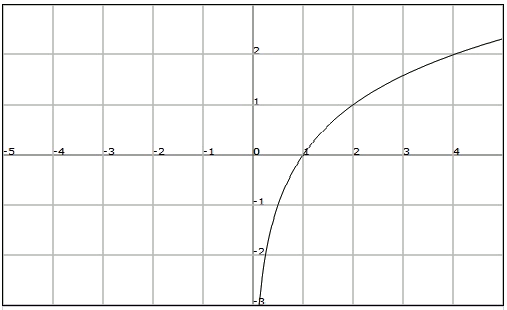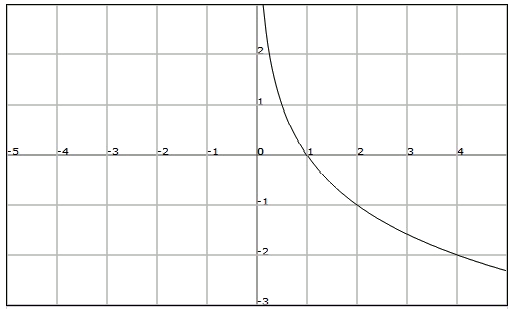# Logarithmic functions

The function that assigns to the independent variable $$x$$ the value of $$f (x) =\log_ax$$ is called logarithmic function of base $$a$$, where $$a$$ is a positive real number other than $$1$$.

We observe that if we apply the log to the exponential function base $$a$$ we obtain the identity function $$\log_a(a^x)=x$$$Similarly it is satisfied that $$a^{\log_ax}=x$$$ Therefore the exponential and logarithmic functions are inverse functions.

## Graph

As in case of the exponential functions, the graph of the logarithmic functions changes if the base is greater to or smaller than $$1$$.

Let's see it with $$f(x)=\log_2x$$ and $$h(x)=\displaystyle \log_{\frac{1}{2}}x$$.

It is remarkable that the logarithmic functions always go thorugh the point $$(1, 0)$$ since any number to the power $$0$$ is $$1$$.

$$f(x)=\log_2x$$$$\displaystyle f(x)=\log_{\frac{1}{2}}x$$## Properties

From its graphic representation we observe that the logarithmic function satisfies the following properties:

• Domain: $$Dom (f) = (0,+\infty)$$
• Image: $$Im (f) = \mathbb{R}$$
• Bounds: It is not bounded.
• Intersection with the axes: It cuts the horizontal axis at $$x = 1$$. It does not cut the vertical axis.
• Continuity: It is continuous in its domain.
• Asimptotes: The straight line $$x = 0$$ is a vertical asimptote.
• If $$a> 1$$: $$\displaystyle \lim_{x \to 0^+} \log_ax=-\infty$$ and $$\displaystyle \lim_{x \to +\infty} \log_ax=+\infty$$
• If $$0 <1$$: $$\displaystyle \lim_{x \to 0^+} \log_ax=+\infty$$ and $$\displaystyle \lim_{x \to +\infty} \log_ax=-\infty$$
• Regularity: It is not periodic.
• Symmetries: It is not symmetric.
• Monotonicity: If $$a> 1$$, the function is strictly increasing. If $$a<1$$, the function is strictly decreasing.
• Relative extrema: It does not have any.
• Injectivity and exhaustivity: It is injective (the images of different points are different), and also it is exhaustive since the image is $$\mathbb{R}$$